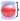# Sphere Volume and Area Calculator

Select Unit### Calculate Sphere Volume?

Calculate the volume of a sphere, Volume = 4/3 * 𝛑 * radius3. If you don't have the radius sphere, you can get it by dividing the diameter by 2.

### Sphere diameter, radius, area ?

If you don't have the radius The radius is half the diameter. Radius = Diameter / 2